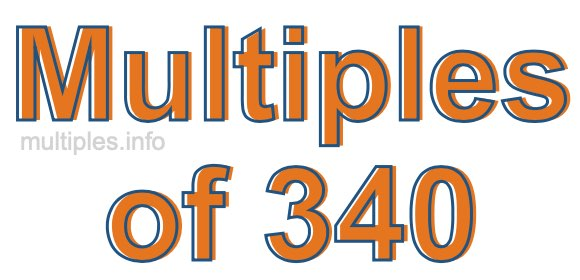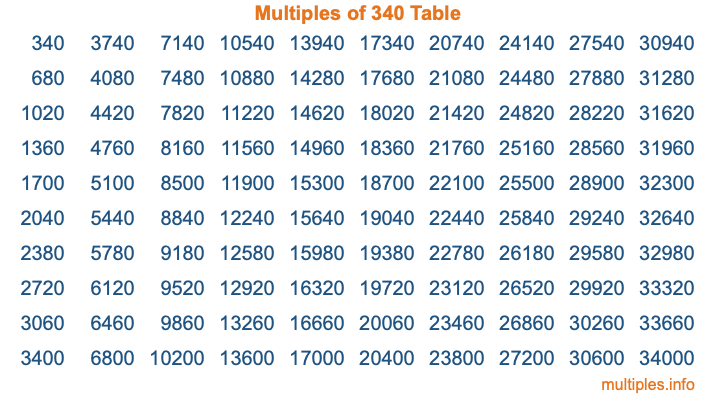Multiples of 340Welcome to the Multiples of 340 page. Here we will first teach you everything you will ever need to know about the multiples of 340, and then give you a study guide summary of everything we taught you to make sure you remember it all. Use this page to look up facts and learn information about the multiples of 340. This page will make you a multiples of three hundred forty expert!

Definition of Multiples of 340
Multiples of 340 are all the numbers that when divided by 340 equal an integer. Each of the multiples of 340 are called a multiple. A multiple of 340 is created by multiplying 340 by an integer.

Therefore, to create a list of multiples of 340, you start with 1 multiplied by 340, then 2 multiplied by 340, then 3 multiplied by 340, and so on for as long as you want. Thus, the list of the first five multiples of 340 is 340, 680, 1020, 1360, and 1700. To see a larger list of multiples of 340, see the printable image of Multiples of 340 further down on this page. We also have a category where you can choose any nth multiple of 340.

Multiples of 340 Checker
The Multiples of 340 Checker below checks to see if any number of your choice is a multiple of 340. In other words, it checks to see if there is any number (integer) that when multiplied by 340 will equal your number. To do that, we divide your number by 340. If the the quotient is an integer, then your number is a multiple of 340.

Is  a multiple of 340?

Least Common Multiple of 340 and ...
A Least Common Multiple (LCM) is the lowest multiple that two or more numbers have in common. This is also called the smallest common multiple or lowest common multiple and is useful to know when you are adding our subtracting fractions. Enter one or more numbers below (340 is already entered) to find the LCM.

Check out our LCM Calculator if you need more details about the Least Common Multiple or if you need the LCM for different numbers for adding and subtraction fractions.

nth Multiple of 340
As we stated above, 340 is the first multiple of 340, 680 is the second multiple of 340, 1020 is the third multiple of 340, and so on. Enter a number below to find the nth multiple of 340.

th multiple of 340

Multiples of 340 vs Factors of 340
340 is a multiple of 340 and a factor of 340, but that is where the similarities end. All postive multiples of 340 are 340 or greater than 340. All positive factors of 340 are 340 or less than 340.

Below is the beginning list of multiples of 340 and the factors of 340 so you can compare:

Multiples of 340: 340, 680, 1020, 1360, 1700, etc.

Factors of 340: 1, 2, 4, 5, 10, 17, 20, 34, 68, 85, 170, 340

As you can see, the multiples of 340 are all the numbers that you can divide by 340 to get a whole number. The factors of 340, on the other hand, are all the whole numbers that you can multiply by another whole number to get 340.

It's also interesting to note that if a number (x) is a factor of 340, then 340 will also be a multiple of that number (x).

Multiples of 340 vs Divisors of 340
The divisors of 340 are all the integers that 340 can be divided by evenly. Below is a list of the divisors of 340.

Divisors of 340: 1, 2, 4, 5, 10, 17, 20, 34, 68, 85, 170, 340

The interesting thing to note here is that if you take any multiple of 340 and divide it by a divisor of 340, you will see that the quotient is an integer.

Multiples of 340 Table
Below is an image of the first 100 multiples of 340 in a table. The table is in chronological order, column by column. The first column has the first ten multiples of 340, the second column has the next ten multiples of 340, and so on.The Multiples of 340 Table is also referred to as the 340 Times Table or Times Table of 340. You are welcome to print out our table for your studies.

Negative Multiples of 340
Although not often discussed or needed in math, it is worth mentioning that you can make a list of negative multiples of 340 by multiplying 340 by -1, then by -2, then by -3, and so on, to get the following list of negative multiples of 340:

-340, -680, -1020, -1360, -1700, etc.

Multiples of 340 Summary
Below is a summary of important Multiples of 340 facts that we have discussed on this page. To retain the knowledge on this page, we recommend that you read through the summary and explain to yourself or a study partner why they hold true.

There are an infinite number of multiples of 340.

A multiple of 340 divided by 340 will equal a whole number.

340 divided by a factor of 340 equals a divisor of 340.

The nth multiple of 340 is n times 340.

The largest factor of 340 is equal to the first positive multiple of 340.

340 is a multiple of every factor of 340.

340 is a multiple of 340.

A multiple of 340 divided by a divisor of 340 equals an integer.

340 divided by a divisor of 340 equals a factor of 340.

Any integer times 340 will equal a multiple of 340.

Multiples of a Number
Here you can get the multiples of another number, all with the same attention to detail as we did for multiples of 340 on this page.

Multiples of
Multiples of 341
Did you find our page about multiples of three hundred forty educational? Do you want more knowledge? Check out the multiples of the next number on our list!